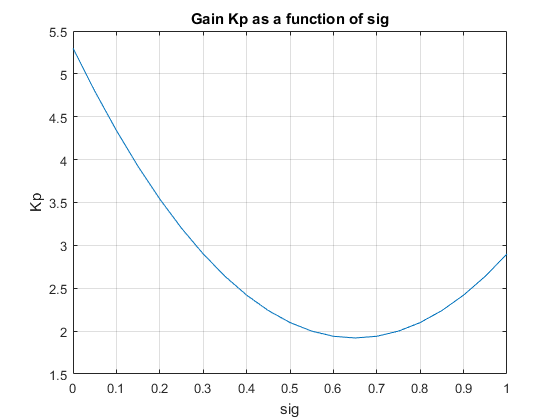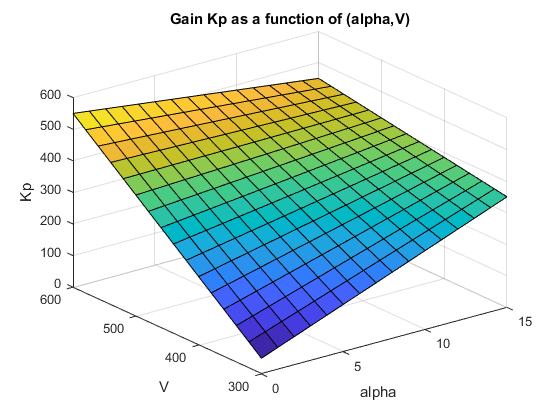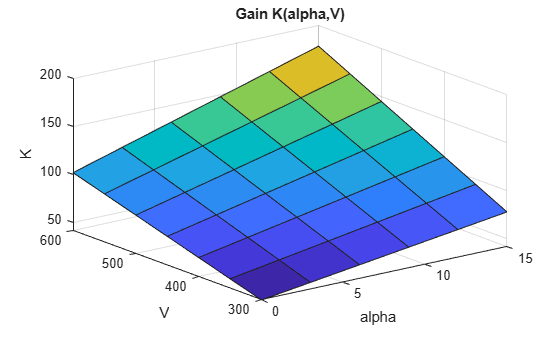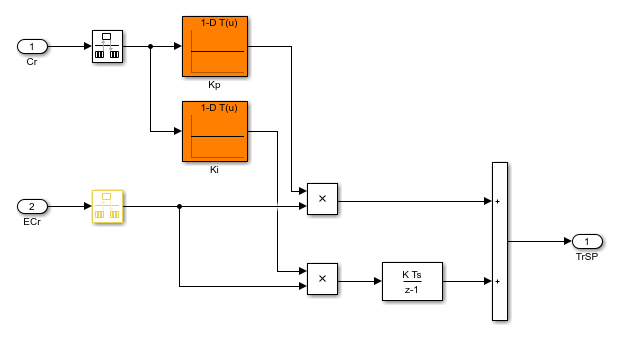## Parameterize Gain Schedules

Typically, gain-scheduled control systems in Simulink® use lookup tables or MATLAB Function blocks to specify gain values as a function of the scheduling variables. For tuning, you replace these blocks by parametric gain surfaces. A parametric gain surface is a basis-function expansion whose coefficients are tunable. For example, you can model a time-varying gain k(t) as a cubic polynomial in t:

k(t) = k0 + k1t + k2t2 + k3t3.

Here, k0,...,k3 are tunable coefficients. When you parameterize scheduled gains in this way, `systune` can tune the gain-surface coefficients to meet your control objectives at a representative set of operating conditions. For applications where gains vary smoothly with the scheduling variables, this approach provides explicit formulas for the gains, which the software can write directly to MATLAB Function blocks. When you use lookup tables, this approach lets you tune a few coefficients rather than many individual lookup-table entries, drastically reducing the number of parameters and ensuring smooth transitions between operating points.

### Basis Function Parameterization

In a gain-scheduled controller, the scheduled gains are functions of the scheduling variables, σ. For example, a gain-scheduled PI controller has the form:

`$C\left(s,\sigma \right)={K}_{p}\left(\sigma \right)+\frac{{K}_{i}\left(\sigma \right)}{s}.$`

Tuning this controller requires determining the functional forms Kp(σ) and Ki(σ) that yield the best system performance over the operating range of σ values. However, tuning arbitrary functions is difficult. Therefore, it is necessary either to consider the function values at only a finite set of points, or restrict the generality of the functions themselves.

In the first approach, you choose a collection of design points, σ, and tune the gains Kp and Ki independently at each design point. The resulting set of gain values is stored in a lookup table driven by the scheduling variables, σ. A drawback of this approach is that tuning might yield substantially different values for neighboring design points, causing undesirable jumps when transitioning from one operating point to another.

Alternatively, you can model the gains as smooth functions of σ, but restrict the generality of such functions by using specific basis function expansions. For example, suppose σ is a scalar variable. You can model Kp(σ) as a quadratic function of σ:

`${K}_{p}\left(\sigma \right)={k}_{0}+{k}_{1}\sigma +{k}_{2}{\sigma }^{2}.$`

After tuning, this parametric gain might have a profile such as the following (the specific shape of the curve depends on the tuned coefficient values and range of σ):Or, suppose that σ consists of two scheduling variables, α and V. Then, you can model Kp(σ) as a bilinear function of α and V:

`${K}_{p}\left(\alpha ,V\right)={k}_{0}+{k}_{1}\alpha +{k}_{2}V+{k}_{3}\alpha V.$`

After tuning, this parametric gain might have a profile such as the following. Here too, the specific shape of the curve depends on the tuned coefficient values and ranges of σ values:For tuning gain schedules with `systune`, you use a parametric gain surface that is a particular expansion of the gain in basis functions of σ:

`$K\left(\sigma \right)={K}_{0}+{K}_{1}{F}_{1}\left(n\left(\sigma \right)\right)+\dots +{K}_{M}{F}_{M}\left(n\left(\sigma \right)\right).$`

The basis functions F1,...,FM are user-selected and fixed. These functions operate on n(σ), where n is a function that scales and normalizes the scheduling variables to the interval [–1,1] (or an interval you specify). The coefficients of the expansion, K0,...,KM, are the tunable parameters of the gain surface. K0,...,KM can be scalar or matrix-valued, depending on the I/O size of the gain K(σ). The choice of basis function is problem-dependent, but in general, try low-order polynomial expansions first.

### Tunable Gain Surfaces

Use the `tunableSurface` command to construct a tunable model of a gain surface sampled over a grid of design points (σ values). For example, consider the gain with bilinear dependence on two scheduling variables, α and V:

`${K}_{p}\left(\alpha ,V\right)={K}_{0}+{K}_{1}\alpha +{K}_{2}V+{K}_{3}\alpha V.$`

Suppose that α is an angle of incidence that ranges from 0° to 15°, and V is a speed that ranges from 300 m/s to 700 m/s. Create a grid of design points that span these ranges. These design points must match the parameter values at which you sample your varying or nonlinear plant. (See Plant Models for Gain-Scheduled Controller Tuning.)

```[alpha,V] = ndgrid(0:5:15,300:100:700); domain = struct('alpha',alpha,'V',V);```

Specify the basis functions for the parameterization of this surface, α, V, and αV. The `tunableSurface` command expects the basis functions to be arranged as a vector of functions of two input variables. You can use an anonymous function to express the basis functions.

`shapefcn = @(alpha,V)[alpha,V,alpha*V];`

Alternatively, use `polyBasis`, `fourierBasis`, or `ndBasis` to generate basis functions of as many scheduling variables as you need.

Create the tunable surface using the design points and basis functions.

`Kp = tunableSurface('Kp',1,domain,shapefcn);`

`Kp` is a tunable model of the gain surface. `tunableSurface` parameterizes the surface as:

`${K}_{p}\left(\alpha ,V\right)={\overline{K}}_{0}+{\overline{K}}_{1}\overline{\alpha }+{\overline{K}}_{2}\overline{V}+{\overline{K}}_{3}\overline{\alpha }\overline{V},$`

where

`$\overline{\alpha }=\frac{\alpha -7.5}{7.5},\text{ }\overline{V}=\frac{V-500}{200}.$`

The surface is expressed in terms of the normalized variables, $\overline{\alpha },\overline{V}\in {\left[-1,1\right]}^{2}$ rather than in terms of α and V. This normalization, which `tunableSurface` performs by default, improves the conditioning of the optimization performed by `systune`. If needed, you can change the default scaling and normalization. (See `tunableSurface`).

The second input argument to `tunableSurface` specifies the initial value of the constant coefficient, K0. By default, K0 is the gain when all the scheduling variables are at the center of their ranges. `tunableSurface` takes the I/O dimensions of the gain surface from K0. Therefore, you can create array-valued tunable gains by providing an array for that input.

`Karr = tunableSurface('Karr',ones(2),domain,shapefcn);`

`Karr` is a 2-by-2 matrix in which each entry is a bilinear function of the scheduling variables with independent coefficients.

### Tunable Gain with Two Independent Scheduling Variables

This example shows how to model a scalar gain K with a bilinear dependence on two scheduling variables. You do so by creating a grid of design points representing the independent dependence of the two variables.

Suppose that the first variable α is an angle of incidence that ranges from 0 to 15 degrees, and the second variable V is a speed that ranges from 300 to 600 m/s. By default, the normalized variables are:

`$x=\frac{\alpha -7.5}{7.5},\phantom{\rule{1em}{0ex}}y=\frac{V-450}{150}.$`

The gain surface is modeled as:

`$K\left(\alpha ,V\right)={K}_{0}+{K}_{1}x+{K}_{2}y+{K}_{3}xy,$`

where ${K}_{0},...,{K}_{3}$ are the tunable parameters.

Create a grid of design points, (α,V), that are linearly spaced in α and V. These design points are the scheduling-variable values used for tuning the gain-surface coefficients. They must correspond to parameter values at which you have sampled the plant.

`[alpha,V] = ndgrid(0:3:15,300:50:600);`

These arrays, `alpha` and `V`, represent the independent variation of the two scheduling variables, each across its full range. Put them into a structure to define the design points for the tunable surface.

`domain = struct('alpha',alpha,'V',V);`

Create the basis functions that describe the bilinear expansion.

`shapefcn = @(x,y) [x,y,x*y]; % or use polyBasis('canonical',1,2)`

In the array returned by `shapefcn`, the basis functions are:

`$\begin{array}{c}{F}_{1}\left(x,y\right)=x\\ {F}_{2}\left(x,y\right)=y\\ {F}_{3}\left(x,y\right)=xy.\end{array}$`

Create the tunable gain surface.

`K = tunableSurface('K',1,domain,shapefcn);`

You can use the tunable surface as the parameterization for a lookup table block or a MATLAB Function block in a Simulink model. Or, use model interconnection commands to incorporate it as a tunable element in a control system modeled in MATLAB. After you tune the coefficients, you can examine the resulting gain surface using the `viewSurf` command. For this example, instead of tuning, manually set the coefficients to non-zero values and view the resulting gain.

```Ktuned = setData(K,[100,28,40,10]); viewSurf(Ktuned)````viewSurf` displays the gain surface as a function of the scheduling variables, for the ranges of values specified by `domain` and stored in the `SamplingGrid` property of the gain surface.

In your Simulink model, you model gain schedules using lookup table blocks, MATLAB Function blocks, or Matrix Interpolation blocks, as described in Model Gain-Scheduled Control Systems in Simulink. To tune these gain surfaces, use `tunableSurface` to create a gain surface for each block. In the `slTuner` interface to the model, designate each gain schedule as a block to tune, and set its parameterization to the corresponding gain surface. For instance, the `rct_CSTR` model includes a gain-scheduled PI controller, the `Concentration controller` subsystem, in which the gains `Kp` and `Ki` vary with the scheduling variable `Cr`.To tune the lookup tables `Kp` and `Ki`, create a tunable surface for each one. Suppose that `CrEQ` is the vector of design points, and that you expect the gains to vary quadratically with `Cr`.

```TuningGrid = struct('Cr',CrEQ); ShapeFcn = @(Cr) [Cr , Cr^2]; Kp = tunableSurface('Kp',0,TuningGrid,ShapeFcn); Ki = tunableSurface('Ki',-2,TuningGrid,ShapeFcn);```

Suppose that you have an array `Gd` of linearizations of the plant subsystem, `CSTR`, at each of the design points in `CrEQ`. (See Plant Models for Gain-Scheduled Controller Tuning). Create an `slTuner` interface that substitutes this array for the plant subsystem and designates the two lookup-table blocks for tuning.

```BlockSubs = struct('Name','rct_CSTR/CSTR','Value',Gd); ST0 = slTuner('rct_CSTR',{'Kp','Ki'},BlockSubs); ```

Finally, use the tunable surfaces to parameterize the lookup tables.

```ST0.setBlockParam('Kp',Kp); ST0.setBlockParam('Ki',Ki);```

When you tune `STO`, `systune` tunes the coefficients of the tunable surfaces `Kp` and `Ki`, so that each tunable surface represents the tuned relationship between `Cr` and the gain. When you write the tuned values back to the block for validation, `setBlockParam` automatically generates tuned lookup-table data by evaluating the tunable surfaces at the breakpoints you specify in the corresponding blocks.

### Tunable Surfaces in MATLAB

For a control system modeled in MATLAB®, use tunable surfaces to construct more complex gain-scheduled control elements, such as gain-scheduled PID controllers, filters, or state-space controllers. For example, suppose that you create two gain surfaces `Kp` and `Ki` using `tunableSurface`. The following command constructs a tunable gain-scheduled PI controller.

`C0 = pid(Kp,Ki);`

Similarly, suppose that you create four matrix-valued gain surfaces `A`, `B`, `C`, `D`. The following command constructs a tunable gain-scheduled state-space controller.

`C1 = ss(A,B,C,D);`

You then incorporate the gain-scheduled controller into a generalized model of your entire control system. For example, suppose `G` is an array of models of your plant sampled at the design points that are specified in `Kp` and `Ki`. Then, the following command builds a tunable model of a gain-scheduled single-loop PID control system.

`T0 = feedback(G*C0,1);`

When you interconnect a tunable surface with other LTI models, the resulting model is an array of tunable generalized `genss` models. The design points in the tunable surface determine the dimensions of the array. Thus, each entry in the array represents the system at the corresponding scheduling variable value. The `SamplingGrid` property of the array stores those design points.

`T0 = feedback(G*Kp,1)`
```T0 = 4x5 array of generalized continuous-time state-space models. Each model has 1 outputs, 1 inputs, 3 states, and the following blocks: Kp: Parametric 1x4 matrix, 1 occurrences. Type "ss(T0)" to see the current value, "get(T0)" to see all properties, and "T0.Blocks" to interact with the blocks.```

The resulting generalized model has tunable blocks corresponding to the gain surfaces used to create the model. In this example, the system has one gain surface, `Kp`, which has the four tunable coefficients corresponding to K0, K1, K2, and K3. Therefore, the tunable block is a vector-valued `realp` parameter with four entries.

When you tune the control system with `systune`, the software tunes the coefficients for each of the design points specified in the tunable surface.

For an example illustrating the entire workflow in MATLAB, see the section "Controller Tuning in MATLAB" in Gain-Scheduled Control of a Chemical Reactor.